# rmm package

The rmm package is getting its own website!

The rmm package provides an interface to fit Bayesian multiple membership multilevel models with endogenized weights using JAGS from within R for a variety of outcomes (linear, logit, conditional logit, Cox, Weibull).

Most multilevel analyses examine how individuals (lower-level units) are affected by their embedding in contextual/aggregate units at a higher level (macro-micro link). The rmm packages uses the multiple membership multilevel model to conceptually reverse this multilevel setup. It allows studying how the effect of units at lower levels propagates to a higher level (micro-macro link).

Previous studies examining micro-macro links either aggregated or disaggregated the data. These approaches obstruct the inherent aggregation problem, cannot separate micro- and macro-level variance, and ignore dependencies among observations, which induces excessive Type-I error. The proposed model is able to overcome these problems by explicitly modeling the aggregation from micro to macro level by including an aggregation function in the regression model. It is a theoretically and statistically sound solution to the study of micro-macro links with regression analysis.

## Model structure

Let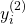be an outcome at level 2, where subscriptindexes level 2 units and superscript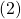indicates that the outcome is located at the second level. Using the multiple membership multilevel model (MMMM) with endogenized weights, we can model this outcome in terms of an aggregated level 1 effect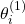and a level 2 effect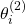:

(1)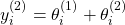The level 2 effect in equation (1) is determined by a systematic component of observed explanatory variables at level 2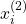with regression coefficients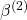and a random component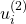:

(2)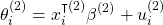The random component at this level is a disturbance term, which is assumed to be normally distributed with a mean of zero and constant variance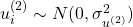. This part of the model represents the conventional single-level model structure.

The aggregated level 1 effectin equation (1) models the aggregation of the effects of level 1 units to the second level. It is determined by a weighted sum of the effect of each level 1 unit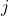on the level 2 outcome in the set of level 1 units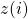embedded in level 2 unit:

(3)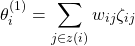That is, subscript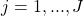indexes level 1 units and the indexing functionreturns all level 1 units that are members of level 2 unit. The multiple membership construct aggregates individual level 1 effects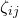by taking their weighted sum with weights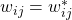for all parties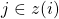and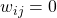for all parties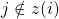.

The individual-level 1 effectsare determined by a systematic component of observed explanatory variables at level 1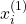with regression coefficients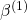and a random component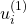, representing the joint impact of unobserved variables:

(4)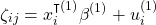The random component at this level is also assumed to be normally distributed with a mean of zero and constant variance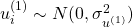.

To examine how the effects of level 1 units propagates to the second level, we endogenize the weights instead of assigning fixed values to each unit:

(5)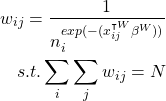where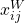are explanatory variables with regression coefficients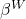,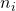is the number of members level 2 unit, and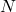equals the total number of observations in the dataset. In this form, the weights are bounded by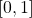.

The weight regression coefficients estimate the impact of explanatory variables on the specific weight of a level 1 unit in its effect on the level 2 outcome. If the weight variables have no impact on the aggregation process, i.e.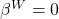, the weights reduce to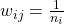(mean aggregation). If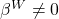, the weights reveal a more complex interplay of level 1 units in their effect on the level 2 outcome. That is, weights will deviate from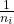and are no longer constant within and between level 2 units. Instead, they depend on.

More details on the model and estimation can be found in this paper draft. I apply the method here.

The rmm package provides a user-friendly way to estimate this model in R.

## Installation of rmm

1. Install JAGS: http://mcmc-jags.sourceforge.net/
2. Install devtools if necessary: install.packages("devtools")
3. Install rmm: devtools::install_github("benrosche/rmm")

In some cases, dependencies are updated before installing the package. If you get the Error: (converted from warning) ..., you can set Sys.setenv("R_REMOTES_NO_ERRORS_FROM_WARNINGS" = "true").

data(coalgov)
rmm(Surv(govdur, earlyterm) ~ 1 + mm(id(gid, pid), mmc(fdep), mmw(w ~ 1/offset(n), constraint=1)) + majority + hm(id=cid, name=cname, type=RE, showFE=F), family="Weibull", monitor=T, data=coalgov)